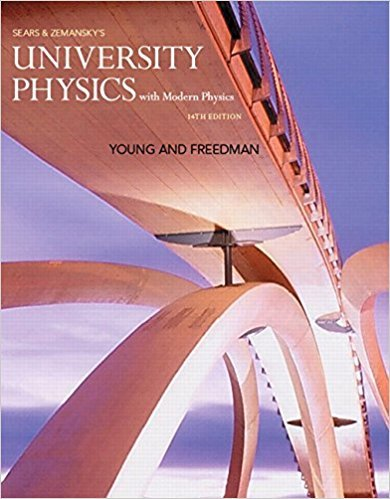×

×

# Solutions for Chapter 6: Work and kinetic energy## Full solutions for University Physics with Modern Physics (1) | 14th Edition

ISBN: 9780321973610Solutions for Chapter 6: Work and kinetic energy

Solutions for Chapter 6
4 5 0 381 Reviews
21
1
##### ISBN: 9780321973610

Chapter 6: Work and kinetic energy includes 121 full step-by-step solutions. University Physics with Modern Physics (1) was written by and is associated to the ISBN: 9780321973610. This textbook survival guide was created for the textbook: University Physics with Modern Physics (1), edition: 14. Since 121 problems in chapter 6: Work and kinetic energy have been answered, more than 187860 students have viewed full step-by-step solutions from this chapter. This expansive textbook survival guide covers the following chapters and their solutions.

Key Physics Terms and definitions covered in this textbook
• //

parallel

• any symbol

average (indicated by a bar over a symbol—e.g., v¯ is average velocity)

• °C

Celsius degree

• °F

Fahrenheit degree